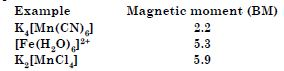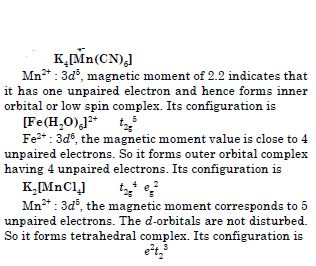# NCERT Solutions for Class 12 Chemistry Chapter 8 - d-and f-Block Elements

Get free NCERT Solutions available on Aasoka to give your best performance for board exams. These solutions are as per the latest CBSE syllabus and designed by subject matter experts. The NCERT Solutions for Class 12 brings you the updated content which makes it easy for students to grab the concepts. It is written in a simple language that can be understood by all and clear all doubts of students.

The chapter “d and f Block Elements” of Chemistry Class 12 makes students familiar with concepts including electronic configuration, variation in ionic, magnetic properties, alloy formation, oxidation states, physical properties, transition elements, properties of important compounds, characteristics of transition metals, and so much more.

##### Question 1:

On what ground can you say that scandium (Z = 21) is a transition element but zinc (Z = 30) is not?

According to definition, transition elements are those which have partially filled d-subshells in their elementary state or in their commonly occurring oxidation states. Scandium (Z = 21) has partially filled d-subshell (3d1) and is therefore, a transition element. On the other hand, zinc (Z = 30) has the configuration 3d104s2. It does not have partially filled d-subshell in its elementary form or in commonly occurring oxidation state (Zn2+ : 3d10). Therefore, it is not regarded as transition element.

##### Question 2:

Why do transition elements exhibit higher enthalpies of atomization ?

The high enthalpies of atomization are due to large number of unpaired electrons in their atoms. Therefore, they have stronger interatomic interactions and hence, stronger bonding between atoms. Thus, they have high enthalpies of atomization.

##### Question 3:

Name a transition element, which does not exhibit variable oxidation states.

Scandium (Z = 21) does not exhibit variable oxidation states.

##### Question 4:

Why is Cr2+ reducing and Mn3+ oxidising when both have d4 configuration?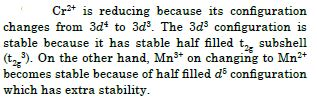##### Question 5:

How would you account for the increasing oxidising power in the series :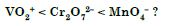This is due to the increasing stability of the lower species to which they are reduced.

##### Question 6:

For the first row transition metals, the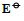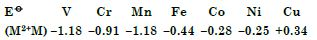Explain the irregularity in the above values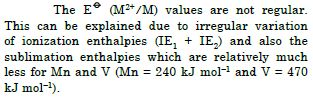##### Question 7: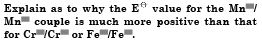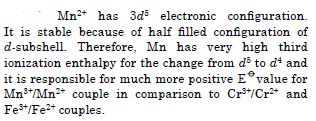##### Question 8:

Calculate the magnetic moment of a divalent ion in aqueous solution if its atomic number is 25.

Divalent ion in aqueous solution having atomic number 25 will have d5 configuration.
No. of unpaired electron = 5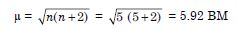##### Question 9:

What is meant by dispropor-tionation of an oxidation state ? Give one example.

When a particular oxidation state becomes less stable relative to other oxidation states, one lower and one higher, it is said to undergo disproportionation. For example, manganese (VI) becomes unstable relative to manganese (VII) and manganese (IV) in acidic solution##### Question 10:

Name a member of lanthanoid series which is well known to exhibit +4 oxidation state.

Cerium (Z = 58)

##### Question 11:

Silver atom has completely filled d-orbitals (4d10) in its ground state. How can you say that it is a transition elements ?

According to definition, transition elements are those which have partially filled d-subshell in their elementary state or in their one of the oxidation states. Silver (Z = 47) can exhibit +2 oxidation state in which it has incompletely filled d-subshell (4d9 configuration). Hence, silver is regarded as transition element.

##### Question 12:

In the series Sc (Z = 21) to Zn (Z = 30), the enthalpy of atomisation of zinc is the lowest i.e., 126 kJ mol–1. Why ?

The high enthalpies of atomization of transition elements is due to the participation of electrons (n–1) d-orbitals in addition to ns electrons in the interatomic metallic bonding. In case of zinc, no electrons from 3d-orbitals are involved in the formation of metallic bonds. Therefore, its enthalpy of atomisation is the lowest. On the other hand, in all other metals of 3d series electrons from d-orbitals are always involved in the formation of metallic bonds.

##### Question 13:

Which of the 3d series of the transition metals exhibits the largest number of oxidation states and why ?

Mn (Z = 25) exhibits the largest number of oxidation states because it has the maximum number of unpaired electrons. Hence, it shows oxidation states from +2 to +7 i.e. +2, +3, +4, +5, +6 and +7.

##### Question 14: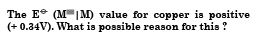The copper has high enthalpy of atomisation and enthalpy of ionization. Therefore, the high energy required to transform Cu(s) to Cu2+ (aq) is not balanced by its low enthalpy of hydration.

##### Question 15:

How would you account for the irregular variation of ionisation enthalpies (first and second) in the first series of transition elements ?

The irregular trend in variation of ionisation enthalpies in the first series of transition elements is because of varying degree of stability of different configurations.

##### Question 16:

Why is the highest oxidation state of a metal exhibited in its oxide or fluoride only ?

Because oxygen and fluorine have small size and high electronegativity, therefore, they can easily oxidise the metal to its highest oxidation state.

##### Question 17:

Which is a stronger reducing agent Cr2+ or Fe2+ and why ?

Cr2+ is a stronger reducing agent than Fe2+. This is because the configuration of Cr2+ changes from d4 to d3 and d3 configuration is stable (t2g3) being half filled t2g level.

##### Question 18:

Calculate the spin only magnetic moment of M2+ (aq) (Z = 27).

The electronic configuration of M2+ (Z = 27)
is M2+ (Z = 27) : 3d7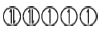It has 3 unpaired electrons. The spin only magnetic moment is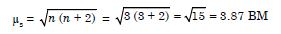##### Question 19:

Explain why Cu+ ion is not stable in aqueous solution ?

Cu+(aq) is not stable in aqueous solution because of its less negative enthalpy of hydration than of Cu2+ (aq).

##### Question 20:

Actinoid contraction is greater from element to element than lanthanoid contraction. Why ?

This is because of relatively poor shielding by 5f electrons in actinoids in comparison with shielding of 4f electrons in lanthanoids.

##### Question 21:

Write down the electronic configuration of :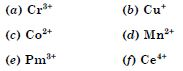(g) Lu2+ (h) Th4+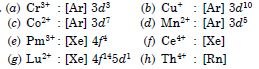##### Question 22:

Why are Mn2+ compounds more stable than Fe2+ towards oxidation to their +3 state ?

Electronic configuration of Mn2+ is [Ar] 3d5 which is half filled and hence it is stable. Therefore, third ionisation enthalpy is very high i.e., third electron cannot be easily removed. In case of Fe2+, the electronic configuration is 3d6. Therefore, Fe2+ can easily lose one electron to acquire 3d5 stable electronic configuration.

##### Question 23:

Explain briefly how +2 state becomes more and more stable in the first half of the first row transition elements with increasing atomic number ?

As we move in the first row transition elements, the sum of IE1 and IE2 increases. As a result reduction potential (E$°$) becomes less and less negative and hence the stability decreases upto first half of the first row elements.

##### Question 24:

To what extent do the electronic configurations decide the stability of oxidation states in the first series of the transition elements? Illustrate your answer with examples.

In a transition series, the oxidation states which result in half filled and completely filled of d-subshell are more stable. For example, in the first transition series, electronic configuration of Mn (Z = 25) is [Ar] 3d5 4s2. It shows oxidation states from +2 to +7 but Mn (II) is most stable because it has stable electronic configuration. Similarly, we can say Zn (Z = 30) having electronic configuration [Ar] 3d104s2 exhibits stable +2 oxidation state because of stable completely filled 3d10 configuration.

##### Question 25:

What may be stable oxidation state of the transition element with the following d-electron configurations in the ground state of their atoms : 3d3, 3d5, 3d8, and 3d4?

The stable oxidation states are predicted on the basis that up to Mn, the maximum oxidation states of stability correspond to sum of s and d-electrons. After Mn, there is decrease in the stability of higher oxidation states.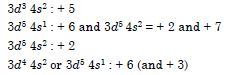##### Question 26:

Name the oxometal anions of the first series of the transition metals in which the metal exhibits the oxidation state equal to its group number.

MnO4 : Oxidation state of Mn = + 7 (Group No = 7)
CrO42–: Oxidation state of Cr = + 6 (Group No = 6)

##### Question 27:

What is lanthanoid contraction ? What are the consequences of lanthanoid contraction?

Lanthanoid contraction. In the lanthanoids there is regular decrease in the size of atoms and ions with increase in atomic number. This decrease of ionic and atomic radii in the lanthanoid elements is called lanthanoid contraction. For example, the ionic radii decrease from Ce3+ (111 pm) to Lu3+ (93 pm). Cause of lanthanoid contraction. As we move through the lanthanoid series, 4f-electrons are being added, one at each step. The mutual shielding effect of electrons is very little, even smaller than that of d-electrons. This is due to the shape of the f-orbitals. The nuclear charge, however, increases by one at each step. Hence, the inward pull experienced by the 4f-electrons increases. This causes a reduction in the size of the entire 4fn shell. The sum of the successive reductions gives the total lanthanoid contraction.

Consequences of lanthanoid contraction. The important consequences of lanthanoid contraction are :

(i) It has very important effect on the relative properties of the elements which follow the lanthanoids with those which proceed the lanthanoids. We know that there is a regular increase in size as we go from Sc to Y to La in group 3. Similarly, we expect normal, increase in size in other groups from Ti $\to$ Zr $\to$ Hf
V $\to$ Nb $\to$ Ta
However after lanthanoids, the increase in radii from second to third transition series vanishes.
Consequently, the pairs of elements Zr—Hf,
Nb—Ta, Mo—W, etc. have almost similar sizes.
Therefore, the properties of these elements are similar.

(ii) There is small but steady increase in the standard electrode potential values (E$°$) for the process :
M3+ + 3e$\to$ M(g)

(iii) The basic strength of oxides and hydroxides decrease with increase in atomic number. Thus, La(OH)3 is most basic and Lu(OH)3 is least basic.

##### Question 28:

What are the characteristics of the transition elements and why are they called transition elements ? Which of the d-block elements may not be regarded as the transition elements ?

The general characteristics of d-block elements are :

1. Nearly all the transition elements have typical metallic properties such have high tensile strength, ductility, malleability, high thermal and electrical conductivity and metallic lustre.
2. Except mercury which is liquid at room temperature, other transition elements have typical metallic structures.
3. They have high melting and boiling points and have higher heats of vaporisation than non-transition elements.
4. They are electropositive in nature.
5. Most of them form coloured compounds.
6. They have good tendency to form complexes.
7. They exhibit several oxidation states.
8. Their compounds are generally paramagnetic in nature.
9. They form alloys with other metals.
10. Most of the transition metals and other compounds are used as catalysts.

They are called transition elements because they represent transition (change) in properties from most electropositive s-block elements to most electronegative p-block elements.

The elements Zn, Cd and Hg are not regarded as transition elements because they donot have partly filled d-subshells in their elementary state or in their commonly occurring oxidation states.

##### Question 29:

In what way is the electronic configuration of the transition elements different from that of the non-transition elements?

The transition elements involve the filling of d-orbitals while the representative elements involve the filling of s or p-orbitals. The general electronic configuration of transition elements is (n–1) d1–10 ns1–2.

On the other hand, the general electronic configuration of representative elements in ns1–2 or ns2 np1–6. Thus, in representative elements, only the last shell is incomplete while in transition elements the last but one shell is also incomplete.

##### Question 30:

What are the different oxidation states exhibited by the lanthanoids ?

The principal oxidation state of lanthanoids is + 3. In addition they exhibit oxidation states of + 2 and + 4.

##### Question 31:

Explain giving reasons :

1. Transition metals and many of their compounds show paramagnetic behaviour.
2. The enthalpies of atomisation of the transition metals are high.
3. The transition metals generally form coloured compounds.
4. Transition metals and their many compounds act as good catalyst.
1. Most of the compounds of transition elements contain unpaired electrons in their (n–1) d-subshells. Therefore, they are paramagnetic in nature and are attracted by the magnetic field. The magnetic character is expressed in terms of magnetic moment. The larger the number of unpaired electrons in a substance, the greater is the paramagnetic character and larger is the magnetic moment. The magnetic moment is expressed in Bohr magneton abbreviated as B.M.
For example, Ti2+ has 2 unpaired electrons and has less magnetic moment than V2+ which has 3 unpaired electrons. Mn2+ has 5 unpaired electrons and has maximum magnetic moment among the divalent transition metal ions because d-subshell can have maximum of 5 unpaired electrons.
2. The high enthalpies of atomization are due to large number of unpaired electrons in their atoms. Therefore, they have stronger interatomic interactions and hence, stronger bonding between atoms. Thus, they have high enthalpies of atomization.
3. Most of the transition metal ions are coloured both in the solid state and in aqueous solutions. The colour of these ions is attributed to the presence of incomplete (n – 1) d-subshell. The electrons in these metal ions can be easily promoted from one energy level to another in the same d-subshell. The amount of energy required to excite the electrons to higher energy states within the same d-subshell corresponds to energy of certain colours of visible light. Therefore, when white light falls on a transition metal compound, some of its energy corresponding to a certain colour, is absorbed causing promotion of d-electrons. This is known as d-d transitions. The remaining colours of white light are transmitted and the compound appears coloured.
For example, hydrated cupric compounds absorb radiations corresponding to red light and the transmitted colour is greenish blue (which is complementry colour to red colour). Thus, cupric compounds have greenish blue colour.
It may be noted that transition metal ions containing completely filled d-orbitals (i.e., d10 configuration) such as Zn2+, Cd2+, Hg2+, Cu+, etc. are generally white. For example, ZnCl2 is white.
4. Some transition metals and their compounds act as good catalysts for various reactions. This is due to their ability to show multiple oxidation states. The common examples are Fe, Co, Ni, V, Cr, Mn, Pt, etc.
The transition metals form reaction intermediates with the substrate by using empty d-orbitals. These intermediates give reaction paths of lower activation energy and therefore, increase the rate of reaction. For example, during the conversion of SO2 to SO3, V2O5 is used as a catalyst. Solid V2O5 absorbs a molecule of SO2 on the surface forming V2O4 and the oxygen is given to SO2 to form SO3. The divanadium tetraoxide is then converted to V2O5 by reaction with oxygen: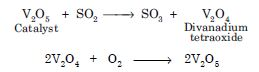2V2O4 + O2 $\to$ 2V2O5
##### Question 32:

What are interstitial compounds ? Why are such compounds well known for transition metals ?

The crystal lattices of transition metals have vacant interstitial sites into which small sized non-metal atoms (H, C, N, B) can easily fit, resulting in the formation of compounds known as interstitial compounds. These are usually non-stoichiometric and are neither typically ionic nor covalent. For example, TiC, Mn4H, Fe3H, TiH1.7, etc. They have chemical properties similar to that of the parent metal but different physical properties such as hardness, electrical conductivity, etc.

##### Question 33:

How is the variability in oxidation states of transition metals different from that of the non-transition metals ? Illustrate with examples.

The variability of oxidation states in transition elements arise because of the participation of (n–1) d-orbitals and ns orbitals. These different oxidation states of transition elements differ by unity. For example, vanadium shows oxidation states of + 2, + 3, + 4 and + 5; manganese shows oxidation states of + 2, + 3, + 4, + 5, + 6 and + 7. On the other hand, some non-transition elements of p-block show variable oxidation states which differ by a unit of two. For example, tin has oxidation states + 2 and + 4, indium has +1 and + 3, etc.

##### Question 34:

Describe the preparation of potassium dichromate from iron chromite ore. What is the effect of increasing pH on a solution of potassium dichromate ?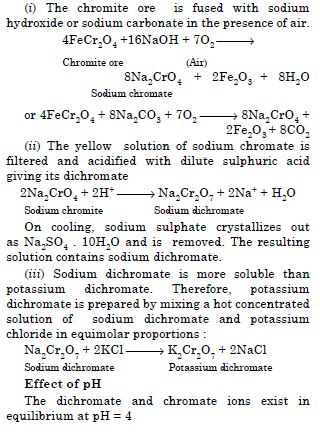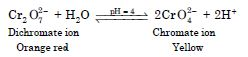On increasing pH (by adding alkali), the H+ ions are used up and according to Le-Chatelier’s principle, the reaction proceeds towards the forward direction producing yellow chromate ions. On decreasing pH (by adding an acid), the reaction shifts towards the backward direction producing the orange dichromate ion.

##### Question 35:

Describe the oxidising action of potassium dichromate and write the ionic equations for its reaction with : (a) iodide (b) iron (II) solution, and (c) H2S.

Potassium dichromate acts as powerful oxidising agent in acidic medium. In the presence of dil. H2SO4, K2Cr2O7 liberates nascent oxygen and, therefore, acts as an oxidising agent.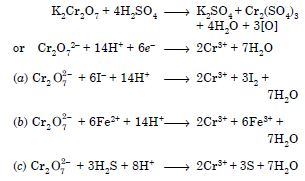##### Question 36:

Describe the preparation of potassium permanganate. How does the acidified permanganate solution reacts with (a) iron (II) ions (b) SO2 and (c) oxalic acid ? Write the ionic equations for the reactions.

Preparation of KMnO4. It is prepared from the mineral pyrolusite (MnO2) by the following steps :
The pyrolusite (MnO2) is fused with caustic potash (KOH) or oxidising agent such as potassium nitrate or potassium chlorate to give a green mass due to the formation of potassium manganate.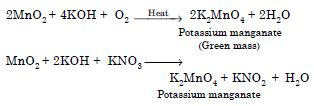The green mass disproportionates in a neutral or acidic solution to give permanganate :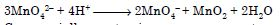Commercially potassium permanganate is prepared by the alkaline oxidative fusion of MnO2 followed by electrolytic oxidation of manganate (VI).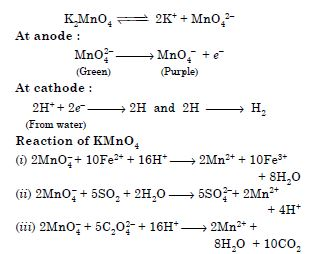##### Question 37:

For M2+|M and M3+|M2+ system the E$°$ values for some metals are as follows: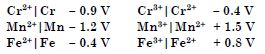Use this data to comment upon :

1. the stability of Fe3+ in acid solution as compared to that of Cr3+ or Mn3+, and
2. the ease with which iron can be oxidised as compared to the similar process for either chromium or manganese metal.

(a) Cr3+|Cr2+ has a negative reduction potential and, therefore, Cr3+ cannot be reduced to Cr2+ i.e., Cr3+ is most stable.
Mn3+|Mn2+ has large +ve value and, therefore, Mn3+ can be easily reduced i.e., Mn3+ is least stable. Fe3+|Fe2+ has small +ve value and, therefore, Fe3+ is more stable than Mn3+ but less stable than Cr3+.

(b) Reduction potential for Mn2+|Mn is most negative and, therefore, it will be most easily oxidized and ease of getting oxidized will be Mn > Cr > Fe.

##### Question 38: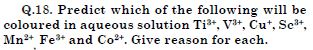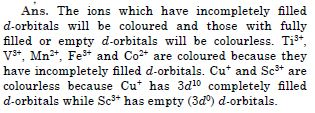##### Question 39:

Compare the chemistry of a actinoids with that of the lanthanoids with special reference to

1. electronic configuration
2. oxidation state
3. atomic and ionic sizes, and
4. chemical reactivity.
1. Electronic configurations. The general electronic configuration of lanthanoids is [Xe]4f1–14 5d0–1 6s2 whereas that of actionoids is [Rn] 5f1–14 6d0–1 7s2. Thus, lanthanoids involve the filling of 4f-orbitals whereas actinoids involve the filling of 5f-orbitals.
2. Oxidation states. Lanthanoids have principal oxidation state of +3. In addition, the lanthanoids show limited oxidation states such as +2, +3 and +4 because of large energy gap between 4f and 5d subshells. On the other hand, actinoids show a large number of oxidation states because of small energy gap between 5f and 6d subshells.
3. Atomic and ionic sizes. Both lanthanoids and actinoids show decrease in size of their atoms or ions in +3 oxidation state. In lanthanoids, the decrease is called lanthanoid contraction and in actinoids, it is called actinoid contraction.
4. Chemical reactivity. Lanthanoids are highly electropositive in nature and they have almost similar chemical reactivity. All the actinoids are also electropositive and have high reactivity. However, in general, actinoids are more reactive (especially in finely divided state) than lanthanoids.
##### Question 40:

How would you account for the following?

1. Of the d4 species, Cr2+ is strongly reducing while Mn(III) is strongly oxidising.
2. Cobalt (II) is stable in a aqueous solution but in the presence of complexing reagents it is easily oxidised.
3. The d1 configuration is very unstable in ions.
1. E values for Cr3+/Cr2+ is negative (– 0.41 V) and for Mn3+/Mn2+ is positive (+1.57V). Thus, Cr2+ can undergo oxidation and, therefore, is reducing agent. On the other hand, Mn(III) can undergo reduction, and therefore, acts as an oxidizing agent.
2. In the presence of complexing agents, cobalt gets oxidised from +2 to +3 state because Co (III) is more stable than Co (II).
3. After loss of ns electrons, d1 electron can easily be lost to give a stable configuration. Therefore, the elements having d1 configuration are either reducing or undergo disproportionation.
##### Question 41:

What is meant by ‘disproportionation’? Give two examples of disproportionation reaction in aqueous solution.

The disproportionation reactions are those in which the same substance gets oxidised as well as reduced. For example,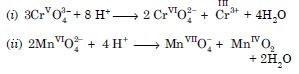##### Question 42:

Which metal in the first series of transition metals exhibits +1 oxidation state most frequently and why?

Copper has electronic configuration [Ar] 3d10 4s1. It can easily lose one (4s1) electron to give stable 3d10 configuration.

##### Question 43:

Calculate the number of unpaired electrons in the following gaseous ions: Mn3+, Cr3+, V3+ and Ti3+. Which one of these is most stable in aqueous solution?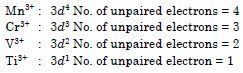##### Question 44:

Give example and suggest reasons for the following features of the transition metal chemistry :

1. The lowest oxide of transition metal is basic, the highest is amphoteric/acidic.
2. A transition metal exhibits highest oxidation state in oxides and fluorides.
3. The highest oxidation state is exhibited in oxoanions of a metal.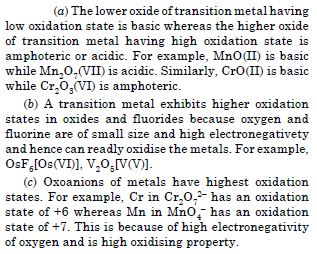##### Question 45:

What are alloys? Name an important alloy which contains some of the lanthanoid metals. Mention its uses.

An alloy is a homogeneous mixture of two or more metals or metals and non-metals.
An important alloy of lanthanoid metals is mischmetal. It contains about 95% lanthanoid metal and about 5% iron and traces of S, C, Ca or Al. It is used in magnesium based alloy to produce bullets, shells and lighter flints.

##### Question 46:

What are inner transition elements ? Decide which of the following atomic numbers are the atomic numbers of the inner transition elements : 29, 59, 74, 95, 102, 104.

The inner transition elements are those in which the last electron enters the f-subshell. These include lanthanoids (from atomic number 58–71) and actinoids (from atomic number 90–103).
Elements having atomic numbers 59, 95 and 102 are inner transition elements.

##### Question 47:

The chemistry of the actinoid elements is not so smooth as that of the lanthanoids. Justify this statement by giving some examples from the oxidation state of these elements.

The chemistry of actinoids is complicated as compared to lanthanoids because actinoids are radioactive and show wide range of oxidation states.
Lanthanoids show limited number of oxidation states, such as +2, +3 and +4 (+3 is the principal oxidation state). This is because of large energy gap between 5d and 4f subshells. On the other hand, actionoids also show principal oxidation state of +3 but show a number of other oxidation states also. For example, uranium (Z = 92) exhibits oxidation states of +3, +4, + 5, + 6, and + 6 and neptunium (Z = 93) shows oxidation states of + 3, + 4, + 5, + 6 and + 7. This is because of small energy difference between 5f and 6d orbitals.

##### Question 48:

Which is the last element in the series of the actionoids ? Write the electronic configuration of this element. Comment on the possible oxidation state of this element.

Last actinoid is lawrencium (Z = 103)
Electronic configuration : [Rn]86 5f14 6d1 7s2
Possible oxidation state : + 3.

##### Question 49:

Which is the last element in the series of the actionoids ? Write the electronic configuration of this element. Comment on the possible oxidation state of this element.

Last actinoid is lawrencium (Z = 103)
Electronic configuration : [Rn]86 5f14 6d1 7s2
Possible oxidation state : + 3.

##### Question 50:

Use Hund’s rule to derive the electronic configuration of Ce3+ ion and calculate its magnetic moment on the basis of ‘spin-only’ formula.

Ce (Z = 58) : [Xe]54 4f1 5d1 6s2
Ce3+ : 4f1
No. of unpaired electrons = 1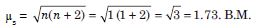##### Question 51:

Name the members of the lanthanoid series which exhibit +4 oxidation states and those which exhibit +2 oxidation states. Try to correlate this type of behaviour with the electronic configurations of these elements.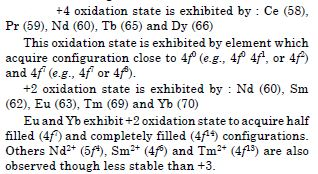##### Question 52:

Write the electronic configurations of the elements with the atomic numbers 61, 91, 101 and 109.

1. Z = 61 : [Xe] 4f5 5d0 6s2
2. Z = 91 : [Rn] 5f2 6d1 7s2
3. Z = 101 : [Rn] 5f13 6d0 7s2
4. Z = 109 : [Rn] 5f14 6d7 7s2
##### Question 53:

Compare the general characteristics of the first series of the transition metals with those of the second and third series metals in the respective vertical columns. Give special emphasis on the following points :

1. Electronic configurations
2. Oxidation states,
3. Ionisation enthalpies and
4. Atomic sizes.
1. Electronic configurations : In the first transition series, 3d orbitals are progressively filled whereas in second and third transition series 4d and 5d orbitals respectively are filled.
2. Oxidation states : Elements show variable oxidation states in all the transition series. Within a column, the maximum oxidation state increases with atomic number. For example in group 8, iron shows common oxidation states of + 2 and + 3 but ruthenium and osmium in the same group form compounds in + 4, + 6 and + 8 oxidation states.
3. Ionisation enthalpies : The ionisation enthalpies of elements of 5d series are higher than those of 3d and 4d series. This is because in 5d series, there are filled 4f-orbitals. The 4f-orbitals have poor shielding effect. As a result, the outer electrons have greater effective nuclear charge acting on the outermost electrons. Therefore, the ionisation enthalpies are higher.
4. Atomic size : The atomic size of 4d series are larger than those of 3d-series. However, the elements of 5d series have nearly the same size as those of 4d series because of lanthanoid contraction.
##### Question 54:

Write down the number of 3d electrons in each of the following ions : Ti2+, V2+, Cr3+, Mn2+, Fe2+, Co2+, Ni2+ and Cu2+. Indicate how would you expect the five 3d orbitals to be occupied for these hydrated ions (octahedral).

(i) Ti2+ : 3d2
For hydrated ions these will be occupied as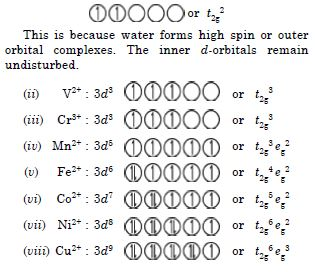##### Question 55:

Comment on the statement that elements of the first transition series possess many properties different from those of heavier transition elements.

The first transition series possess many properties different from those of heavier elements such as :

1. In first transition series, lower oxidation state is more stable whereas in heavier transition elements higher oxidation states are more stable.
2. The ionisation enthalpy of heavier elements (5d series) is quite higher than those of first transition series.
3. Metal-metal bonding is most common in heavier transition metals but very less in first transition series.
4. The elements of first transition series donot form complexes with higher coordination numbers 7 and 8 which is common with heavier elements.
##### Question 56:

What can be inferred from the magnetic moment values of the following complex species?